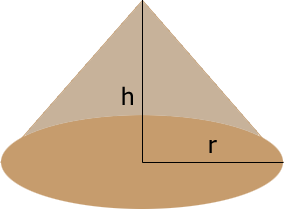SEARCH HOMEMath Central Quandaries & QueriesQuestion from Perry: I have a pile of topsoil that is 42ft long and 16ft tall shaped like a cone how many yards of topsoil do I have.Hi Perry,

The volume of a cone that is $h$ feet high and has a base radius of $r$ feet is

$\frac13 \pi\; r^2 h \mbox{ cubic feet.}$If your conical pile has a height of 16 feet and the radius of the base is 42/2 = 21 feet then the volume is

$\frac13 \pi \; r^2 h= \frac13 \pi \times 21^2 \times 16 = 7,389 \mbox{ cubic feet.}$

There are $3 \times 3 \times 3 = 27$ cubic feet in a cubic yard so that's 7,389/27 = 274 cubic yards.

PennyMath Central is supported by the University of Regina and The Pacific Institute for the Mathematical Sciences.